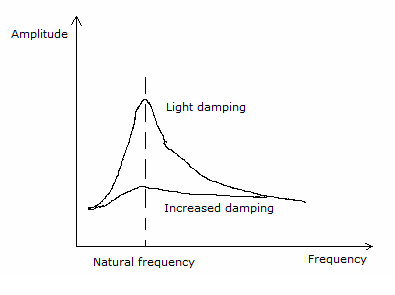# Forced oscillations and resonance

## 4.3.1 State what is meant by damping.

Damping is a dissipating force that is always in the opposite direction to the direction of motion of the oscillating particle. As work is being done against the dissipating force energy is lost. Since energy is proportional to the amplitude, the amplitude decreases exponentially with time.

## 4.3.2 Describe examples of damped oscillations.

Under damped -where there is  a small dissipating force and a fraction of the total energy is removed after every oscillation and hence the amplitude decreases.

Critical damped - this is when there is an intermediate dissipating force and the system reaches equilibrium position as fast as possible without oscillating.

Over damped - there is a large dissipating force and the system takes longer to reach equilibrium position than critical damping. There are no oscillations in over damping.

## 4.3.3 State what is meant by natural frequency of vibration and forced oscillations.

Natural frequency - the frequency at which the system vibrates when in motion

Forced oscillation- when an external force is applied to the original frequency causing a change in the frequency of the oscillation.

## 4.3.4 Describe graphically the variation with forced frequency of the amplitude of vibration of an object close to its natural frequency of vibration.

The amplitude of the forced oscillation depends on  comparative values of the natural frequency and the driving frequency. In addition it also depends on the amount of damping presentFigure 4.3.1 - Variation with forced frequency of the amplitude of vibration of an object

## 4.3.5 State what is meant by resonance.

Resonance is when the natural frequency of a system is equal to the frequency of an external force. This results in oscillating and an increase in amplitude.

## 4.3.6 Describe examples of resonance where the effect is useful and where it should be avoided.

Useful

Quartz oscillators, microwave

Should be avoided

Generators and vibrations in machinery.